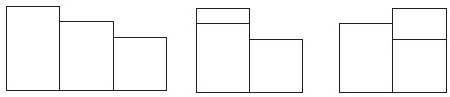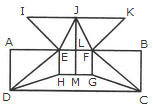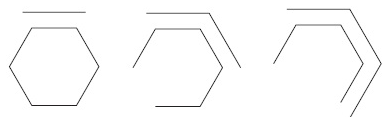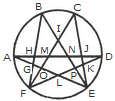# REASONING - Online Test

Q1. What comes next?Explaination / Solution:

The rectangle starting on the left is moving from left to right one place at a time at each stage.

Q2. Find the number of triangles in the given figure.Explaination / Solution:

The figure may be labelled as shown.The simplest triangles are AHG, AIG, AIB, JFE, CJE and CED i.e. 6 in number.

The triangles composed of two components each are ABG, CFE, ACJ and EGI i.e. 4 in number.

The triangles composed of three components each are ACE, AGE and CFD i.e. 3 in number.

There is only one triangle i.e. AHE composed of four components.

Therefore, There are 6 + 4 + 3 + 1 = 14 triangles in the given figure.

Q3.
Directions: Below is given a passage followed by several possible inferences which can be drawn from the facts stated in the passage. You have to examine each inference separately in the context of the passage and decide upon its degree of truth or falsity.
Note: Each of the five questions has only one distinct answer, ie, no two questions can have the same answer. If
you get the same answer for more than one question, consider both again and decide which one of the two would more definitely be that answer and in the same way review the others also.
Cardiovascular disease is so prevalent that virtually all businesses are likely to have employees who suffer from,
or may develop, this condition. Research shows that between 50-80% of all people who suffer a heart attack are able to return to work. However, this may not be possible if they have previously been involved in heavy physical work. In such cases, it may be possible to move the employee to lighter duties, with appropriate retraining where necessary. Similarly Difficult pressure, stressful work, even where it does not involve physical activity, should also be avoided. Human resource managers should be aware of the implications of job roles for employees with a cardiac condition.
Employees who suffer from cardiovascular diseases are mostly
Explaination / Solution:

Research- to work, Line shows that given statement is definitely false.

Q4.
Directions: Study the following information and answer the questions.
Ten people sitting in two parallel rows having five people each in such a way that there is equal distance between adjacent persons. In row-1, A,B,C,D and E are seated (not necessarily in the same order) and all of them are facing south. In row-2 P,Q,R,S and T are seated (not necessarily in the same order) and all of them are facing north. Therefore, in the given seating arrangement each member seated in a row faces another member of the row.

T sits third to the right of Q. P sits second to the left of T. The person facing P sits on immediate right of B. Only one person sits between B and D. A is not an immediate neighbor of B. Only two people sit between A and C. Neither B nor A faces S.
Who among the following facing B?
Explaination / Solution:
No Explaination.

Q5. Which is the odd one out?
Explaination / Solution:

It has three white and one black on the left and three black and one white on the right. The rest are the opposite way round.

Q6. Find the minimum number of straight lines required to make the given figure.Explaination / Solution:

The figure may be labelled as shown.The horizontal lines are IK, AB, HG and DC i.e. 4 in number.

The vertical lines are AD, EH, JM, FG and BC i.e. 5 in number.

The slanting lines are IE, JE, JF, KF, DE, DH, FC and GC i.e. 8 is number.

Thus, there are 4 + 5 + 8 = 17 straight lines in the figure.

Q7. Employees suffering from cardiovascular diseases are unable to handle stressful situations.
Explaination / Solution:

Similarly- be avoided. Shows that given statement is probably true.

Q8.
Directions: Study the following information and answer the questions.
Ten people sitting in two parallel rows having five people each in such a way that there is equal distance between adjacent persons. In row-1, A,B,C,D and E are seated (not necessarily in the same order) and all of them are facing south. In row-2 P,Q,R,S and T are seated (not necessarily in the same order) and all of them are facing north. Therefore, in the given seating arrangement each member seated in a row faces another member of the row.

T sits third to the right of Q. P sits second to the left of T. The person facing P sits on immediate right of B. Only one person sits between B and D. A is not an immediate neighbor of B. Only two people sit between A and C. Neither B nor A faces S.
Which of the following statements is TRUE regarding D ?
Explaination / Solution:
No Explaination.

Q9. What comes next?Explaination / Solution:

The inner hexagon is being dismantled one side at a time working anti clockwise, while the outer hexagon is being constructed one side at a time working clockwise.

Q10. Find the number of triangles in the given figure.Explaination / Solution:

The figure may be labelled as shown.The simplest triangles are AGH, GFO, LFO, DJK, EKP, PEL and IMN i.e. 7 in number.

The triangles having two components each are GFL, KEL, AMO, NDP, BHN, CMJ, NEJ and HFM i.e. 8 in number.

The triangles having three components each are IOE, IFP, BIF and CEI i.e. 4 in number.

The triangles having four components each are ANE and DMF i.e. 2 in number.

The triangles having five components each are FCK, BGE and ADL i.e. 3 in number.

The triangles having six components each are BPF, COE, DHF and AJE i.e. 4 in number.

Total number of triangles in the figure = 7 + 8 + 4 + 2 + 3 + 4 = 28.